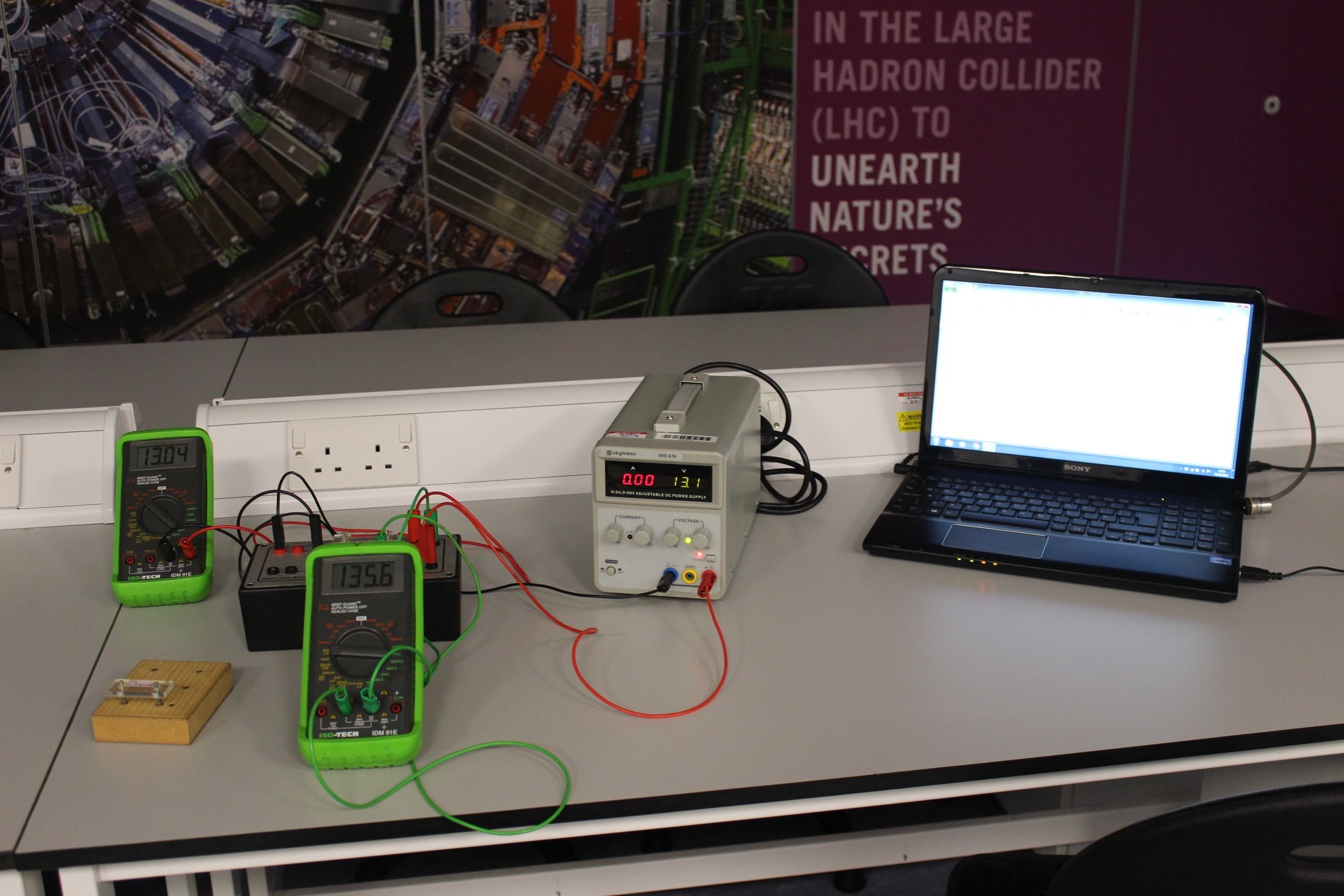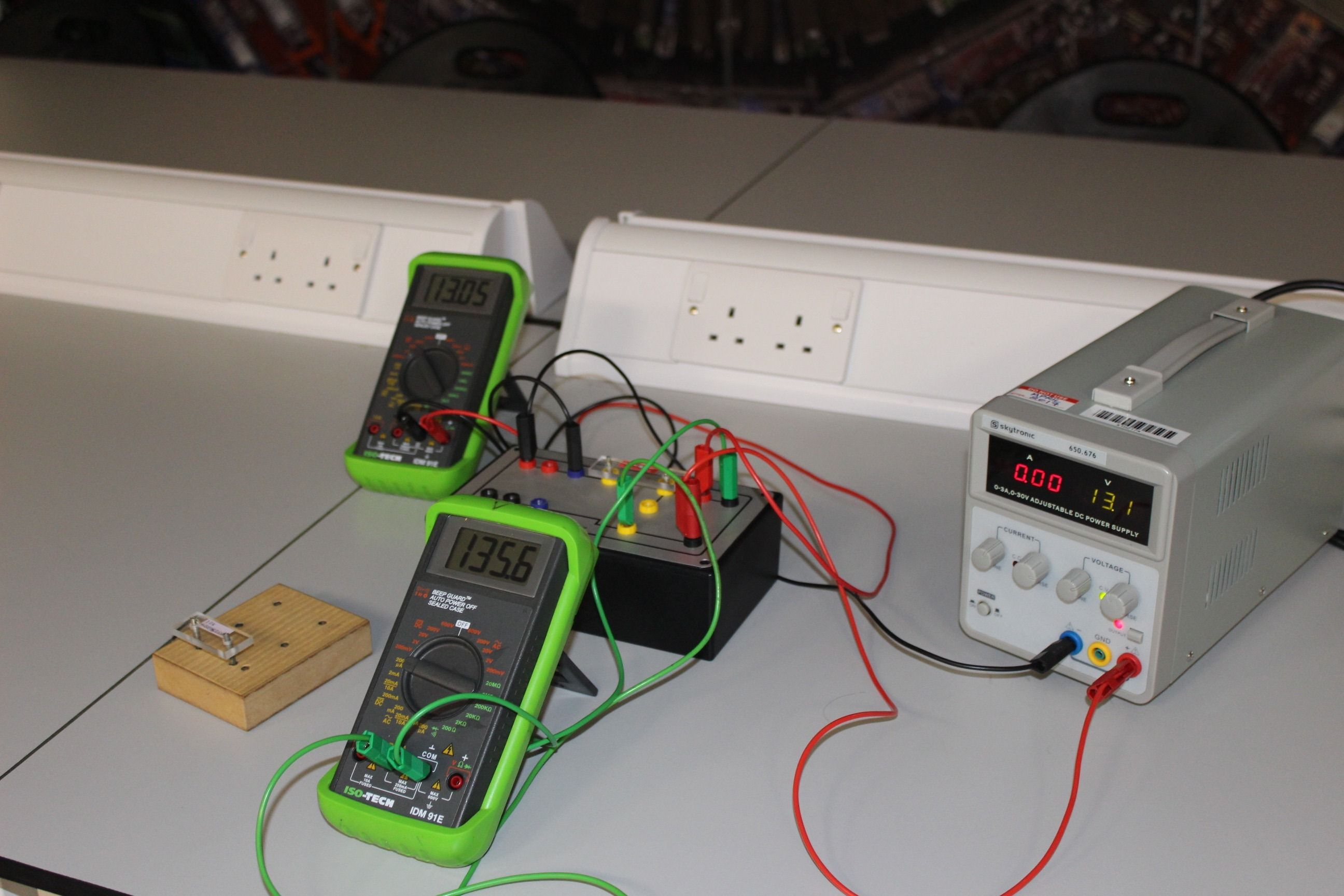Equipment given in sessionWhere to plug in the wires on the circuit block

## Circuits 1

### Introduction

Nearly everything we do involves circuits of some sort. Turning on lights, making a cup of tea, watching television are everyday examples. Circuits can be very simple, such as a light bulb, or extremely complicated and involving many different components. In order to understand how any circuit works we need to understand how electric current flows through it. This experiment is about understanding simple electronic circuits. It introduces you to the measurement of voltage, current and resistance in direct current circuits and experimental strategies based on error analysis will be introduced. You will also verify Ohm's law.

### Script

The script for this experiment can be found in the lab script book or on DUO.